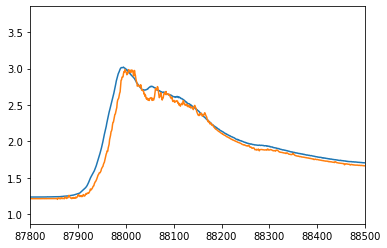#### Notebooks

##### Taiao Case 4-

Author:

##### Content:

We load the required libraries used to train the LSTM

``````import tensorflow as tf
import tensorflow.keras as keras
import tensorflow.keras.backend as K
from tensorflow.keras.layers import LSTM
from tensorflow.keras.layers import Dense
from tensorflow.keras.preprocessing.sequence import TimeseriesGenerator
import pandas as pd
import numpy as np
import matplotlib.pyplot as pyplot``````

The flood prediction dataset has been encapsulated in the packages below and can be loaded using the code below

``````import taiao.dataset.river as data
import taiao.visualization.river as visualiser
import taiao.model.river as models``````
``pd.DataFrame(xTrain).tail()``

We set the hyper-parameters and load the dataset and create the keras data generator

``````forecast=12
lookback=288
xTrain = data.x('train',forecast)
yTrain = data.y('train',forecast)
xTest = data.x('test',forecast)
yTest = data.y('test',forecast)
trainGen=TimeseriesGenerator(xTrain,yTrain,length=lookback,batch_size=3)
testGen=TimeseriesGenerator(xTest,yTest,length=lookback,batch_size=1)

featureCount=xTrain.shape
depth=2``````

Here we define the model and train the output

``````model = models.LSTM(depth,featureCount,lookback, optimizer="adam")
history = model.fit(trainGen,validation_data=testGen, epochs=1).history
model.save('3DLookBack_3hr_forecast_rmse')``````
``134315/134322 [============================>.] - ETA: 0s - loss: 0.0027``

We now evaluate the model

``````model.evaluate(testGen)
trainPredict = model.predict(trainGen)
testPredict = model.predict(testGen)``````
``172522/172522 [==============================] - 469s 3ms/step - loss: 9.9911e-04``

and plot the output for the flood event of interest

``````pyplot.plot(yTest[lookback:])
pyplot.plot(testPredict)
pyplot.xlim((87800,88500))
pyplot.show(block=False)``````

We do a dump of the csv file comparing the predicted river level to the actual river level

``````CsvTemp=np.concatenate([yTest[lookback:].reshape(-1,1),testPredict],axis=1)
np.savetxt('3D_Prediction_3hr_rmse.csv',CsvTemp,delimiter=',')``````